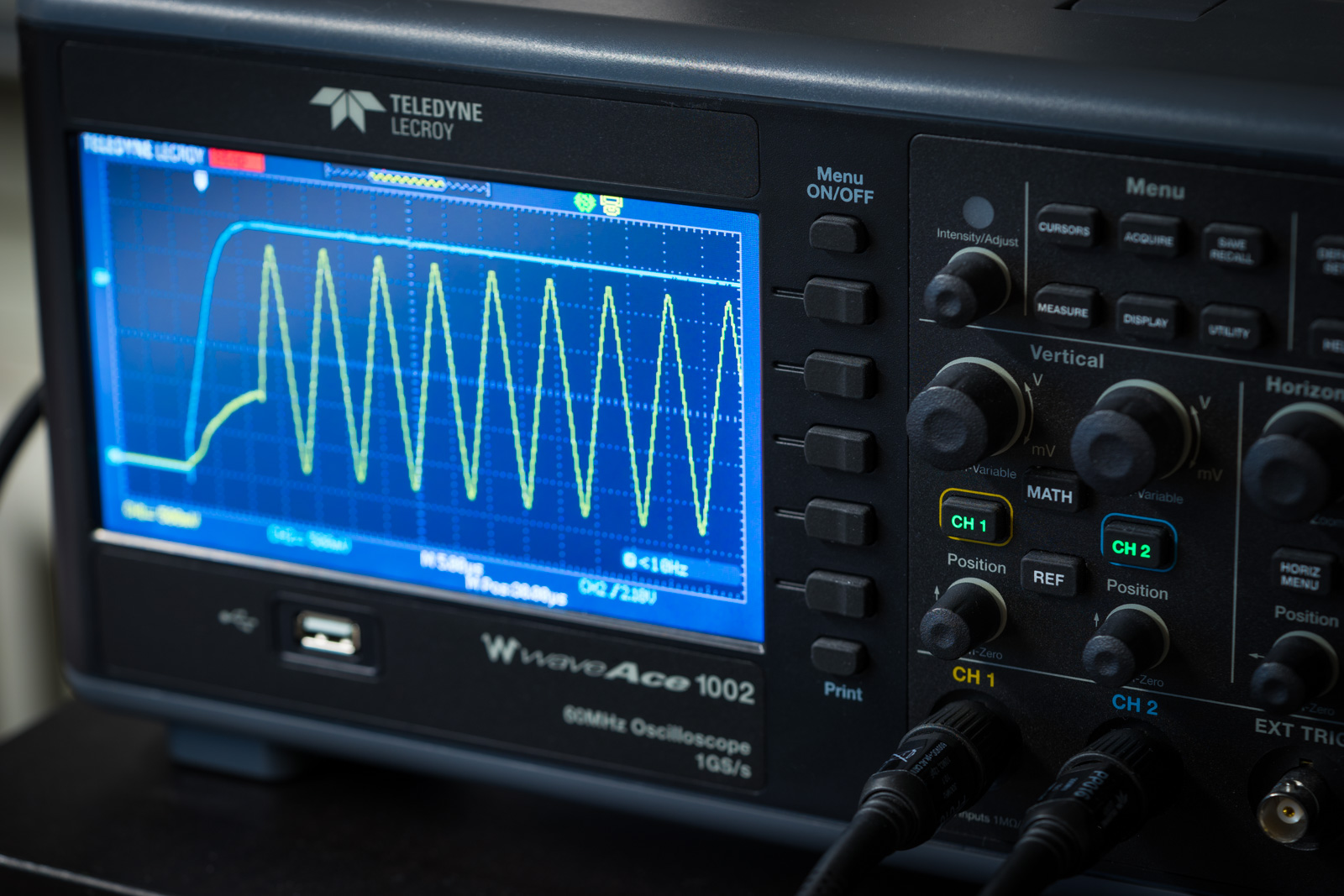# How to Design a Cheap Plant Watering Sensor (Part 2)

This is the second part of the meta-tutorial, where I talk about designing a cheap plant watering sensor. If you did not already read the first part, please do it now. It contains a lot information about constraints and decisions made which lead to this point.

The first part ended with step 11, building a working prototype with the selected key components. So let us start with the next steps in this journey.

# Step 12: Analyse and Measure the Prototype

Never forget why you actually built a prototype. It is your tool to verify all assumptions you made in the design phase. To do this you need the right measuring instruments.

## The Power Usage

I start measuring the current of the circuit. This will show if my assumptions about the battery life will be true. For this test I use a multimeter which has a good resolution measuring in the µA range. The multimeter I use is the Testo 760-3 which is not a very well known brand. Multimeters are usually really poor at measuring low currents on low voltages, so let us see if this will work.

I also use a Fluke 114, but this one has no current measurement. It is sometimes very handy to have two multimeters, one to measure the voltage and a second one to measure the current.

For the first test I program the MCU to do all the tests in a loop and connect the power directly to the second part of the circuit. Now the power is always on and I can measure the current used by the MCU while doing the measurement.Just running, doing the measurement of the oscillator, the second part of the circuit uses at maximum ~1.2mA. This measurement phase should be as short as possible. Later we will analyse the timing.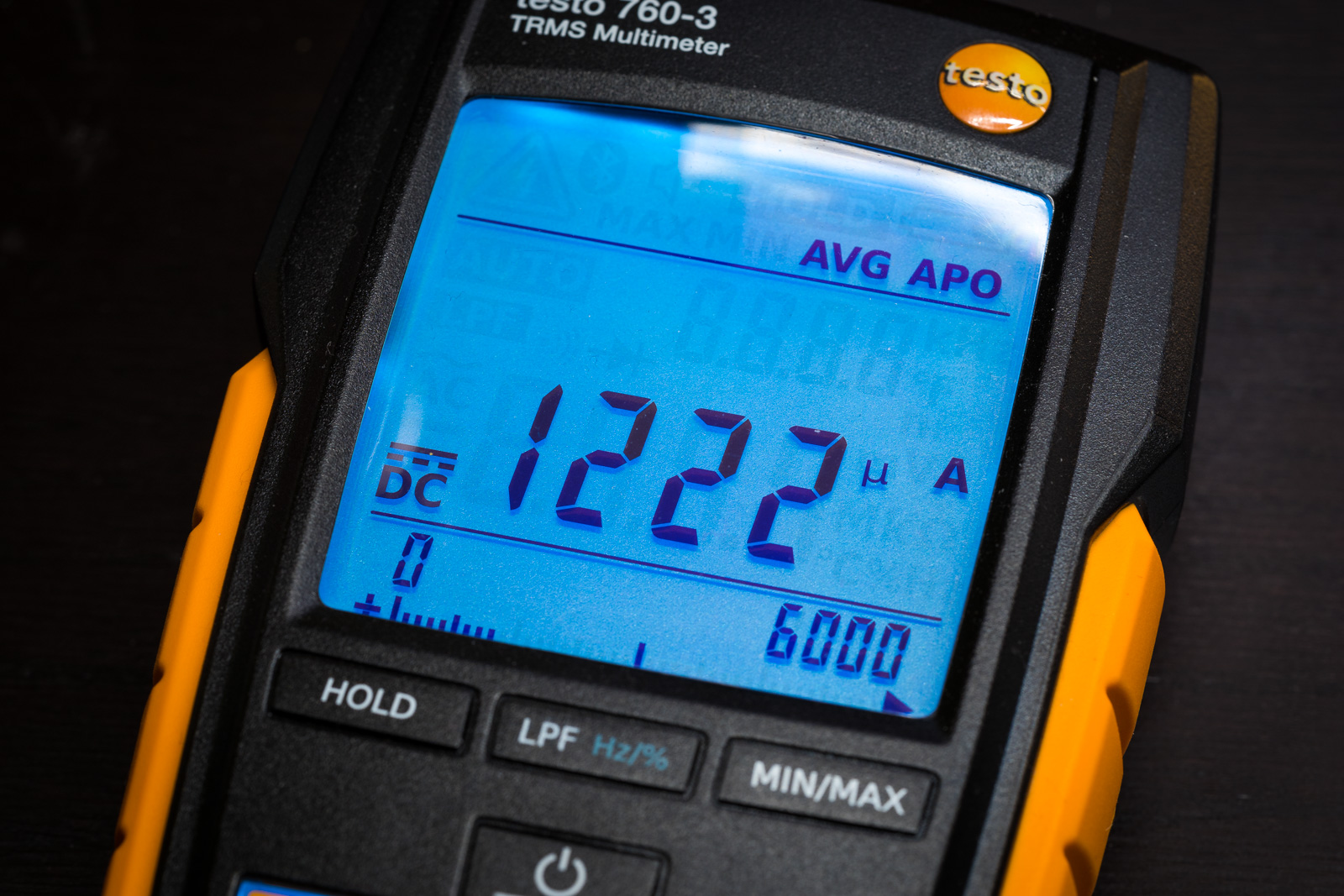The average is just slightly lower, so there are no huge current variations. It seems 1.2mA is a good value to work with.

The multimeter introduces a voltage drop of just 20mV, this is ok. Because I power the circuit from my lab power supply, I could compensate even greater voltage drops.

Next I connect the power to the system timer to check if the power usage more or less matches the specs. The current for the timer should be somewhere in the range of 50nA, but there is also a small current leak from the used P-channel MOSFET.The multimeter displays a value somewhere around 1µA, this can not be right. The specification for the ON/Fairchild BSS84 MOSFET, IDSS maximum is 15µA. It seems this multimeter is not usable to measure this low currents at this low voltages. I will have to investigate this later in detail.

So let us assume 1µA would be right and do the math:$\frac{225\textrm{mAh}}{1\mu\textrm{A}}=225000\textrm{h}=9375\textrm{days}\approx 25\textrm{years}$

This is still a very good value, and at the current point of the project nothing to be concerned about. I will have to look into a device to measure currents in the nano ampere range properly.

## The LED is a Current Hungry Monster

While the LED is on, the current usage will rise up to 20mA. This is the point where the device uses its most power. The coin cell can usually deliver not much more than 20mA current. This will be the absolute limit and the time the LED is on should be reduced to the absolute minimum. Because in normal operation no signal is sent, this should be not that often.

A 40ms flash from the LED is already well visible. Let us do some math. First calculate the required energy for a single flash:$20\textrm{mA}\times 40\textrm{ms}=0.22\mu\textrm{A}/\textrm{h}$

The LED flashes every 10 seconds, 360 times per hour for 40ms:$360\times 0.22\mu\textrm{A}/\textrm{h} = 79.2\mu\textrm{A}/\textrm{h}$

If the user does not stop the blinking LED by watering the plant or resetting the device, the battery will last…$\frac{225\textrm{mAh}}{79.2\mu\textrm{A}/\textrm{h}} \approx 2841\textrm{h} \implies 118\textrm{days}$

These are four months of flashing non-stop, this should never happen if the device is used as intended. Otherwise the plant will die together with the battery. 🙂

## Measure the Oscillator Timing

A very critical part is the timing of the oscillator. Not the generated frequency, the actual oscillation start is the very interesting part. Some oscillators need a while until the oscillation gets stable. And some need some time until the oscillation starts. This is the critical time which the firmware has to wait until a proper measurement can be done.

So it is time to use the Oscilloscope to do some measurements. I am sure it will not disappoint me, as the Testo 760-3 did at the low current measurement. 🙂I use the Teledyne LeCroy WaveAce 1002. It is a (relatively) cheap oscilloscope, but well suited for all my low frequency measurements. I like the simple export of measured data and screenshots and also some nice built-in analyses which take advantage of the digital measurements.

For the first measurement I connect one probe (blue, CH2) to the oscillator power. So every time the oscillator gets some power, I will see the edge of this power rise and can use it as trigger. The second probe (yellow, CH1) is connected to the oscillator output. I set the trigger point to 2.1V. This is the minimum voltage where everything has to work.

I start single capture and repeat it around ten times, checking if I get a reproducible and stable result. Then I repeat it at different voltages to see if the voltage has an influence on the result. In this case the result stays more or less the same without significant changes.As you can see (one grid division is 5µs), the oscillation starts already after 7µs. The first edge of the oscillation looks a little bit different, but starting from the second edge, the oscillation is very stable. Taking a measurement after 30µs would get a proper result. This is very quick – a microcontroller is not ready in this time. A microcontroller will need way longer to start up.

## Measure the Microcontroller Start-Up Time

To measure the start-up time of the microcontroller, I program a special firmware. It will just initialise all registers and then flash the LED for some milliseconds. Now I connect the first probe (yellow, CH1) to the LED. The second probe is still attached to the power and used as trigger.

As before, I do multiple measurements and also at different voltages, to see if I get different results. Same here like the oscillator, the start-up timing of the microcontroller is stable and always the same.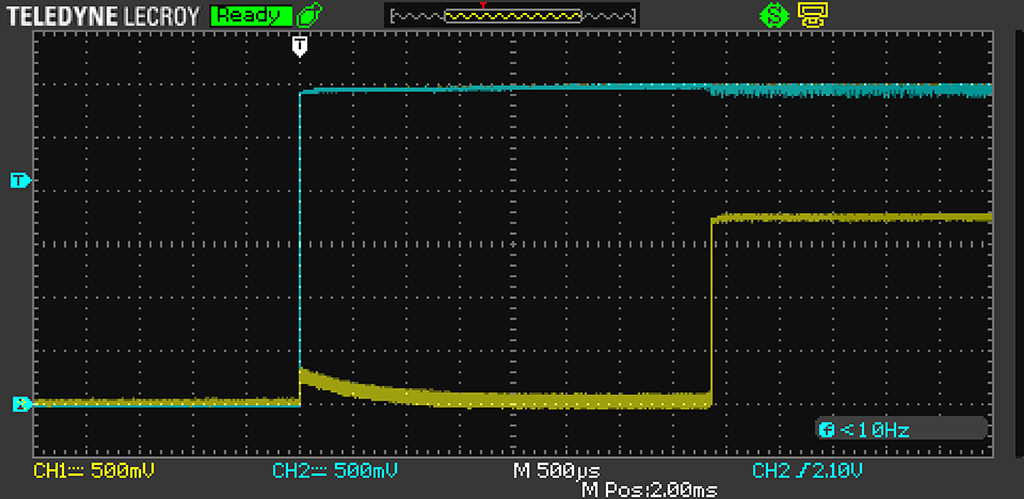First, please note the different scale. One grid unit is now 500µs, 100 times more as for the oscillator measurement. The yellow curve is the measurement at the LED anode. As you can see, the microcontroller requires ~4ms to start. This should be the fastest setting of the microcontroller. It should be:$\begin{matrix}1\textrm{CK} = \frac{1s}{9.6\textrm{MHz}} = 0.104\mu\textrm{s}\\ \text{Startup} = 6\textrm{CK} + 14\text{CK} = 20\times 0.104\mu\textrm{s} = 2.08\mu\textrm{s}\end{matrix}$

Plus a few cycles to set the registers to the initial values. Seems there is some artificial delay added to the start-up. This is something I have to investigate, but even 4ms start-up time is reasonable fast and will be no problem for the device.

## Measure the Voltage DividerThe last check is for the simple voltage divider which is used to check the battery status. A voltage divider is required, because the microcontroller has an internal voltage reference with 1.1V. Obviously I can not use the VCC pin power as reference, because it will always be the same as the measured value.

If I use the 1.1V internal voltage reference, I have to reduce the voltage for the measurement below this value, which is done using this voltage divider.

First let us do some math to check what we should expect:$\begin{matrix}V_o=V_i\times\left(\frac{R_2}{R_1+R_2}\right)\\3\textrm{V}\times\left(\frac{15\textrm{k}\Omega}{47\textrm{k}\Omega+15\textrm{k}\Omega}\right)=725.81\textrm{mV}\end{matrix}$

This would be the ideal value for a 3V supply voltage. Because I use resistors with 5% tolerance in the prototype, I have to calculate the absolute minimum and maximum values to see if the result matches the expectations.$\begin{matrix}R_1=47\textrm{k}\Omega\pm5\%&R_{1min}=44.65\textrm{k}\Omega&R_{1max}=49.35\textrm{k}\Omega\\ R_2=15\textrm{k}\Omega\pm5\%&R_{2min}=14.25\textrm{k}\Omega&R_{2max}=15.75\textrm{k}\Omega\end{matrix}$$\begin{matrix}V_{max}=3\textrm{V}\times\left(\frac{15.75\textrm{k}\Omega}{44.65\textrm{k}\Omega+15.75\textrm{k}\Omega}\right)=782.3\textrm{mV}\\ V_{min}=3\textrm{V}\times\left(\frac{14.25\textrm{k}\Omega}{49.35\textrm{k}\Omega+14.25\textrm{k}\Omega}\right)=672.2\textrm{mV}\\ V_\Delta=110.1\textrm{mV}\end{matrix}$

Now I measure the values in the prototype. First I measure the supply voltage, which is 3.064V. This is quite close to the calculated reference. Now I measure the voltage in the middle of the divider, here I get 842mV. This is way out of the expected range – now I remove each resistor and measure them.$\begin{matrix}R3=46.50\textrm{k}\Omega\\ R4=\underline{\mathbf{17.76}}\textrm{k}\Omega\end{matrix}$

Great! I silently trash the defect resistor R4 and replace it with one which measures 14.96kΩ. Now I measure 741mV, which is very close to the calculated value.

This is everything which can be verified at the moment. It is still only a prototype and the values can change slightly if everything is on a PCB. All this measurements are important to verify if the analog parts of the circuit work as expected.

# Step 13: Check the Firmware Inputs

After we verified the analog parts, it is time to see if we can actually use the analog signals as input for the firmware.

## Verify the Button Input

I start with the simplest input, the button (S1) which is connected to PB3. Here I can use the signal LED to test this input. I rewrite the firmware like this:

void setStatusLed(bool enabled)
{
if (enabled) {
PORTB |= _BV(DDB1);
} else {
PORTB &amp;amp;= ~_BV(DDB1);
}
}

bool isButtonPressed()
{
return ((PINB &amp;amp; _BV(PINB3)) != 0);
}

int main(void)
{
setupHardware();

while (1) {
setStatusLed(isButtonPressed());
}
}


I only show the relevant code here. You can see the method setupHardware() is missing. I also remove all comments from the code. In a later part of this tutorial I will focus on the firmware and talk about all this things in detail.

Now I can simply press the button and it lights up the signal LED. Works perfect!

## Verify the Voltage Divider Input

Next I check the input from the voltage divider. The ATtiny13 microcontroller has no really usable outputs, like serial lines or similar. The best way to deal with this is using the step by step debugger which is available using the debugWire connection.

I rewrite the code to store the output of the ADC into a volatile variable. This way I can set a breakpoint and output this value.

uint16_t getVoltageValue()
{
uint16_t values = 0u;
const uint8_t sampleCount = 8u;
for (uint8_t i = 0; i &amp;lt; sampleCount; ++i) {
_NOP();
}
values += value;
}
return (values / sampleCount);
}

volatile uint16_t currentVoltageValue = 0;

int main(void)
{
setupHardware();

while (1) {
currentVoltageValue = getVoltageValue();
delayMs(100);
}
}


In AmtelStudio I set a breakpoint at the line “delayMs(100)” and edit this breakpoint. Here I configure an action which prints the variable “currentVoltageValue”.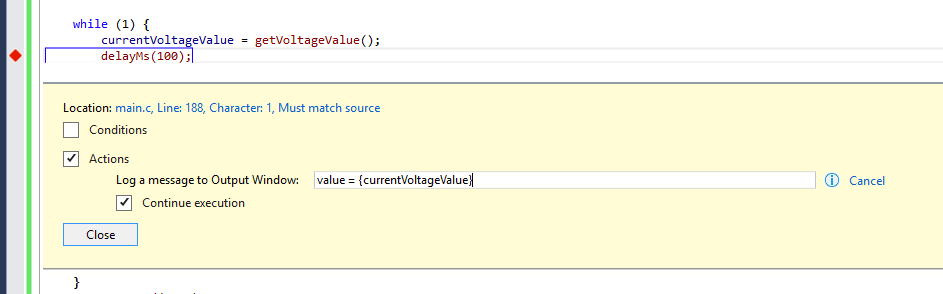Now I can watch the value of my ADC function live: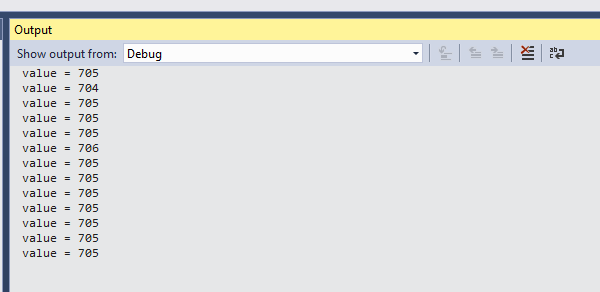If I change the supply voltage from 3V down to 2.1V I can watch how the value goes down to ~500 and back to ~705. This works as expected. Later, on a real PCB prototype, I will have to experiment with a real coin cell for realistic values and see if the currently visible fluctuations (±1) are still there (probably noise from the oscillator) and see how to get a stable value.

## Verify the Oscillator Input

Here I rewrite the code like this:

uint8_t getOscillatorFrequency()
{
TCNT0 = 0;
TIFR0 = _BV(TOV0);
delayMs(1);
uint8_t result = TCNT0;
if ((TIFR0 &amp; _BV(TOV0)) != 0) {
result = 0xff;
}
return result;
}

volatile uint16_t currentOscillatorFrequency = 0;

int main(void)
{
setupHardware();
while (1) {
currentOscillatorFrequency = getOscillatorFrequency();
delayMs(100);
}
}


I use the same method as before. Now I can watch the oscillator value live in the output window. If I touch the sensor, the frequency goes down to 50 back up to 130. Great, seems everything is working and the input are perfectly usable.

# Step 14: Design a Prototype PCB

Now I verified my circuit using the prototype and found no large issues, I design a prototype PCB. This prototype should be close to the final device with all the knowledge I already have. It is important to test the circuit of the device with the real components directly soldered on a PCB.

I only design the “head” part of the sensor, without the part to stick into the soil. For the first tests I will use the PCBs I already have. After designing this prototype, I will order a few boards from OSH Park. This is a really cheap option if you only need a few boards.

After ordering the boards, I have to wait until they arrive before I can continue with the project.

The microcontroller has to be prepared before it is soldered to the board. I also design a small programmer device where I can prepare the microcontroller with the required fuse bits.

# Epilogue

In a few weeks I will publish the next part, which will further describe the work from the PCB prototype to the final device.

Have fun!

## 6 thoughts on “How to Design a Cheap Plant Watering Sensor (Part 2)”

1.E says:

Very interesting. I need to make a comment though on the resistive method that has ‘almost only disadvantages’. That is true, but it sure has some advantages as well, namely cheap and simple. I have made both resistive and capacitive watering sensors and am well aware that corrosion is a big ‘disadvantage’ in resistive sensors, but that is rather easy to curb by letting the sensor only measure for a short while (miliseconds), say every 4 hrs. Sure, corrosion is inevitable but I have one such sensor that is going strong for 3 seasons.
Now obviously it may not be a good idea to use pcb tracks for resistive measurements (Most of the chinese webshop watering sensors do that), but if you want just one device that you can stick in the soil with no fuss can use galvanized nails that stick in a connector on a PCB.

Having said that… your observations are all very valid, your design and end product are really great and make a great tutorial, so I am definitely not criticizing, and I am really happy with the capacitive sensors I made (though of a different design than you, using a simple schmitt-trigger oscillator. But for those who want something fast and cheap, don’t completely rule out the resistive measuring.

Anyway, I will go through the theory of yr publication a bit deeper and see if you had the same findings as I did.
Great stuff

1.Lucky Resistor says:

Thank you very much for this comment. You have a point here about the resistive measurement method. I changed the article and added a new point “Good: Simples and cheapest measurement method” to the list. You are also right, I just considered PCB tracks, which corrode quickly away, large nails will last much longer of course.

2.Julien says:

Thank you ! This is very interesting and very well written ! This is the kind of articles I would like to read more often on DIY websites. I stay here waiting for the next parts =)

3.themba202 says:

Interesting approach and well executed! I like how you factor in all aspects (cost, ease of use, battery life,…) from the start.

One quick tip: you don’t need the voltage divider to measure the battery voltage. The avr line of micro’s offers a neat feature where it can measure it’s internal 1.1V reference compared to Vcc instead of measuring some external voltage compared to the internal reference. Since you know the reference voltage you can then back-calculate the Vcc.
Have a look at, for instance, this: https://provideyourown.com/2012/secret-arduino-voltmeter-measure-battery-voltage/

1.Lucky Resistor says:

Thank you very much! The used ATtiny13A does not has this feature, but it would be actually great to free one pin for other tasks.

4.themba202 says:

It seems that you are right. (I actually checked the attiny85 datasheet, (wrongfully) assuming that if one attiny has it then all would..). It seems that you are out of luck with the attiny13. All the others attiny’s seem to have it (24,25, 44, 45, 84, 85) (except the attiny10 and friends)… They are pin compatible so if you want the extra pin you can still change uC…

This site uses Akismet to reduce spam. Learn how your comment data is processed.